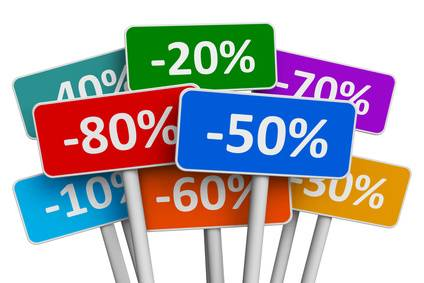# The department store where you work is having a sale. Every item is to be marked down 30%. What will the sale price of a \$53 sweater be?

30% means 30/100 = 0.3

0.3 * \$53

= \$15.90

======Subtract this number from \$53.

thanked the writer.

The final sale price for the item will be \$37.10.

I've explained how I worked that out step=by-step below:

30% can look complicated to work out but actually, any multiple of ten is an easy calculation that can be done in your head.To get the hang of percentages, start with an easy to handle number like 10%... For 10% all you need to do is move the decimal point one place to the left so 10% of \$53 (think of it as \$53.00 as this will be easier to visualise) would be \$5.30.

Now, to get the 30% you are actually looking for, all you have to do is times it by however many more tens it is. So in this case it is 30% and therefore three times our 10% calculation.

Three fives are fifteen, 3 times thirty is ninety so therefore 30% of \$53 is \$15.90.

Then just take the percentage amount (\$15.90) from the main price (\$53), and you are left with a sale price of \$37.10 - bargain! :-)

thanked the writer.